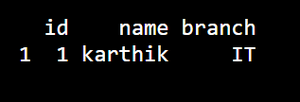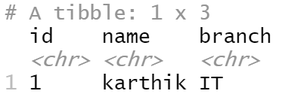GeeksforGeeks App
Open AppBrowser
Continue

## Related Articles

In this article, we will see how to convert the named vector to Dataframe in the R Programming Language.

Method 1:

Generally while converting a named vector to a dataframe we may face a problem. That is, names of vectors may get converted into row names, and data may be converted into a single column. So we need to convert the vector into a list then convert the list into a dataframe.

First, we will convert the vector into a list using as.list( ) method and passed it to data.frame( ) method in order to convert the vector into dataframe.

Example:

## R

 `vector1 = ``c``(1, ``"karthik"``, ``"IT"``)``names``(vector1) = ``c``(``"id"``, ``"name"``, ``"branch"``)`` ` `df = ``data.frame``(``as.list``(vector1))``print``(df)`

Output :Method 2: Using tibble library.

In tibble library there is a method called as_tibble( ) function. In order to use as_tibble( ) we need to install tibble library. To install package we can use install.packages( ) function by passing package name as parameter.

syntax : variable = as_tibble (as.list(vector))

Example:

## R

 `library``(tibble)``vec1 = ``c``(``"1"``, ``"karthik"``, ``"IT"``)``names``(vec1) = ``c``(``"id"``, ``"name"``, ``"branch"``)`` ` `df=``as_tibble``(``as.list``(vec1))``print``(df)`

Output:My Personal Notes arrow_drop_up